# Direction Sense MCQ - 2

## 20 Questions MCQ Test Reasoning Aptitude for Competitive Examinations | Direction Sense MCQ - 2

Description
Attempt Direction Sense MCQ - 2 | 20 questions in 15 minutes | Mock test for LR preparation | Free important questions MCQ to study Reasoning Aptitude for Competitive Examinations for LR Exam | Download free PDF with solutions
QUESTION: 1

Solution:
QUESTION: 2

### If 3’o clock in a  watch.If the minute hand points towards the North east then the hour hand will point towards

Solution: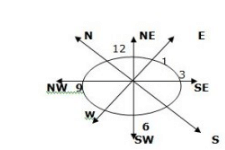QUESTION: 3

### ​ Kiran started walking towards east .After walking 10m,he took a right turn and walked 10m.He then took a left turn and walked 30m and again took a left turn and walked 40m.Now Kiran how far and in which direction from the starting point

Solution: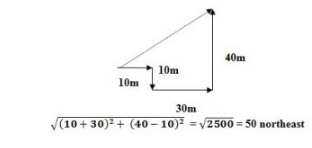QUESTION: 4

P walks 8m to the south ,then he turn to his left and walks 15m then he turn to his right and walk 12m again he turns to his right and walk 15m and turn right and stopped how far and in which direction from the starting point ?

Solution: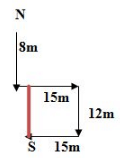QUESTION: 5

If southeast become North, North-east become West and so on.What will be the West ?

Solution:

Option ( a) is the right answer.

"South -east "

If south-east becomes North and north east becomes west, therefore,the whole figure moves 135 degree. Hence, West will bw south east. see, actual figure is rotating 135 degree anticlockwise. So, whenwest will be retated by same degree anticlockwise. It will hold the place of south east.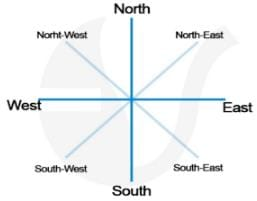QUESTION: 6

Sakthi starts from her house and walks 3km towards north, then she turns right and walks 2km and then turn right and walks 5km then turns and walks 2km and then again turns right and walks 2km.Which direction is she facing now?

Solution: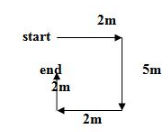QUESTION: 7

A, B, C , D ,E , F, G and H are sitting around a circular table in the same order for a discussion at equal distances .Their positions are clockwise.If G sits in the north ,then what will be the position of D?

Solution: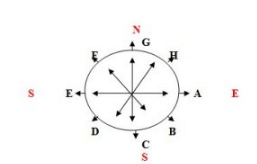From the diagram, we can see that the position of D is South- west.

QUESTION: 8

Manik walks 4m towards east then turn to his right and walks 4m and then turn to his left and walk 5m.Again turning to her left he walks 4m and stopped.What is the shortest distance between the starting point and ending point ?

Solution: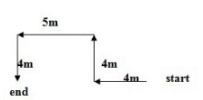QUESTION: 9

Kamali walks a distance of 3m towards North then turns to her left and walk for 2m.She turns left and walks for 3m.At this point she turns to his left and walk for 3m.How many metres is she from the starting point ?

Solution: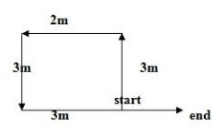QUESTION: 10

Sahil walked 20m toward North, took a left turn and walked 10m.He again took a left turn and walked for 20m.How far and in which direction is he from the starting point ?

Solution: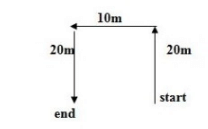QUESTION: 11

Sarita leaves from home .She walk 25m in North west direction and then 25m in south west direction.Next she walks 25m in south east direction.Finally she turn toward her house.Now in which direction she was moving ?

Solution: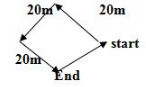QUESTION: 12

One day afternoon Arun and Mani were sitting in a garden and facing each other.Mani’s shadow fall exactly towards Arun’s right, Which direction was Mani facing ?

Solution: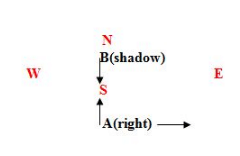QUESTION: 13

P is 15m to the west of Q. R is 13m to the south of Q and 5m to the east of S. T is 20m to the north of S.T is 10m to the east of U…….

Q.

In which direction U with respect to P

Solution: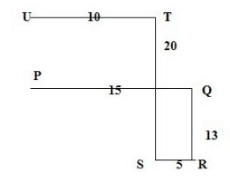QUESTION: 14

P is 15m to the west of Q. R is 13m to the south of Q and 5m to the east of S. T is 20m to the north of S.T is 10m to the east of U…….

Q.

If A is 7m to the west of T, then which of the following will be nearest point to A.  00

Solution:
QUESTION: 15

P is 15m to the west of Q. R is 13m to the south of Q and 5m to the east of S. T is 20m to the north of S.T is 10m to the east of U…….

Q.

In which direction R with respect to P

Solution:
QUESTION: 16

Point W is 7m towards the West of point X. Point X is 3m towards the North of point Y. Point Z is 4m towards West of point Y……………

Q. A person walks 3m towards North from point Z and reaches point P . How far and towards which direction will he have to walk to reach point W ?

Solution: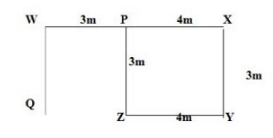QUESTION: 17

Point W is 7m towards the West of point X. Point X is 3m towards the North of point Y. Point Z is 4m towards West of point Y……………

Q.

If a person walks 3m towards South from point W and reaches point Q, which of the following 2 points would fall in straight line including Q

Solution:
QUESTION: 18

Point W is 7m towards the West of point X. Point X is 3m towards the North of point Y. Point Z is 4m towards West of point Y……………

Q.If a person walks 3m towards South from point W and reaches point Q, which of the following 2 points would fall in straight line including Q

Solution:
QUESTION: 19

Point W is 7m towards the West of point X. Point X is 3m towards the North of point Y. Point Z is 4m towards West of point Y……………

Q. What is the position of X with respect to P

Solution:

X is towards the east wrt to P

QUESTION: 20

Vivek travelled 12kms West, then he turned left and travelled 8kms. Then he turned left and travelled 12kms. How far was Vivek from starting point ?

Solution: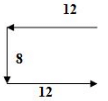Use Code STAYHOME200 and get INR 200 additional OFF Use Coupon Code